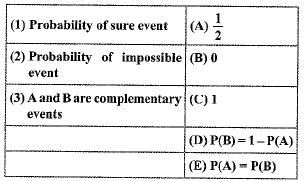Courses

# RS Aggarwal Test: Probability

## 10 Questions MCQ Test Mathematics (Maths) Class 10 | RS Aggarwal Test: Probability

Description
This mock test of RS Aggarwal Test: Probability for Class 10 helps you for every Class 10 entrance exam. This contains 10 Multiple Choice Questions for Class 10 RS Aggarwal Test: Probability (mcq) to study with solutions a complete question bank. The solved questions answers in this RS Aggarwal Test: Probability quiz give you a good mix of easy questions and tough questions. Class 10 students definitely take this RS Aggarwal Test: Probability exercise for a better result in the exam. You can find other RS Aggarwal Test: Probability extra questions, long questions & short questions for Class 10 on EduRev as well by searching above.
QUESTION: 1

Solution:
QUESTION: 2

### A card is drawn from a deck of 52 cards. The event E is that card is not an ace of hearts. The number of outcomes favourable to E is

Solution:

Total number of outcomes =52

we have only 1 ace of hearts , so the number of favourable outcomes of E ,

where E is card not an ace of hearts = 51.

QUESTION: 3

### Which of the following cannot be the probability of an event?

Solution:
QUESTION: 4

Match the columns:Solution:

Probability facts.

QUESTION: 5

If P(A) denotes the probability of an event A, then

Solution:
QUESTION: 6

When a die is thrown once, the probability of getting an odd number less than 3 is

Solution:
QUESTION: 7

The probability of getting a bad egg in a lot of 400 is 0.035. The number of bad eggs in the lot is

Solution:
QUESTION: 8

A coin is tossed 1000 times and 640 times a ‘head’ occurs. The empirical probability of occurrence of a head in this case is

Solution:

P = 640/1000 = 0.64

QUESTION: 9

Two coins are tossed simultaneously. The probability of getting atmost one head is

Solution:

Two coin tossed
Total sample space = {HH, HT, TH, TT}
Sample space for getting atmost one head = {TT, TH, HT}
P(s) = 3/4

​

QUESTION: 10

A card is selected from a deck of 52 cards. The probability of being a red face card is

Solution: